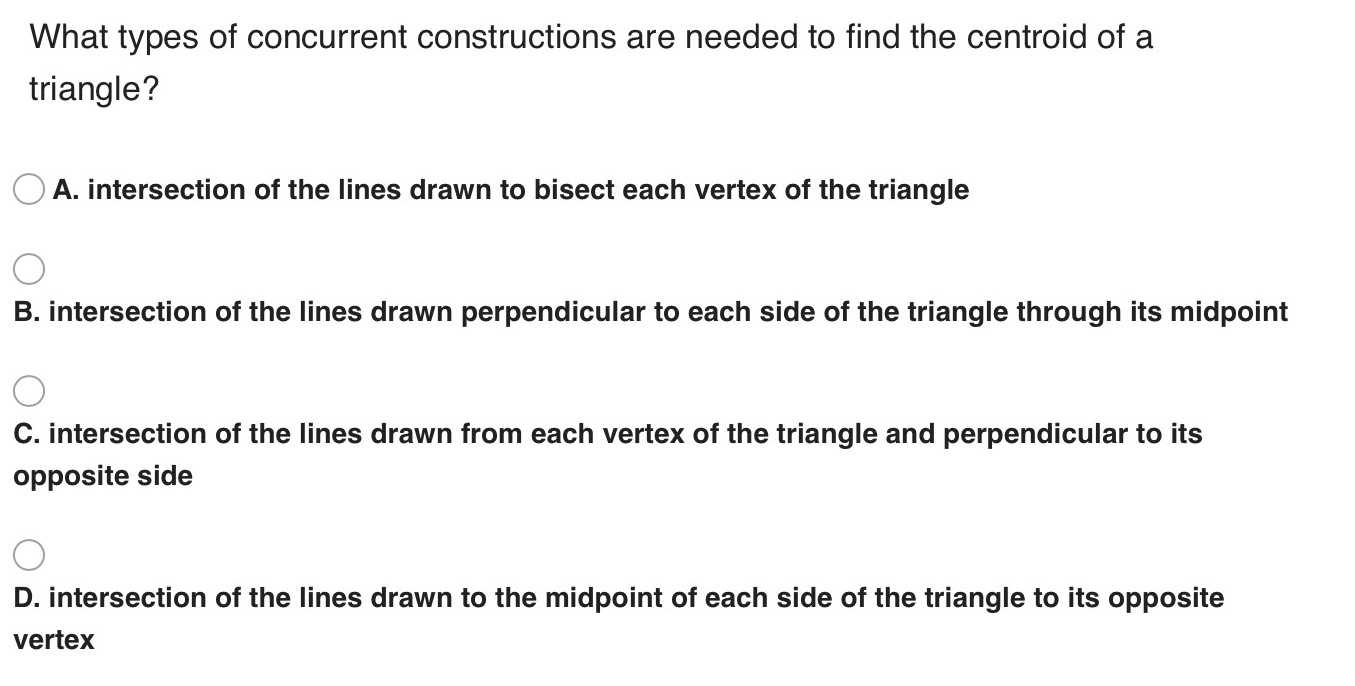### Still have math questions?

Trigonometry
QuestionWhat types of concurrent constructions are needed to find the centroid of a triangle?

A. intersection of the lines drawn to bisect each vertex of the triangle

B. intersection of the lines drawn perpendicular to each side of the triangle through its midpoint

C. intersection of the lines drawn from each vertex of the triangle and perpendicular to its opposite side

D. intersection of the lines drawn to the midpoint of each side of the triangle to its opposite vertex